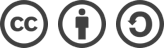## SDUT 2778 小明的花费预算（二分答案）解题报告

2016-02-18 551 次浏览

## 题面

Time Limit: 1000ms Memory limit: 65536K

5 3
3
2
9
4
1

9

lwn

## 解题思路

#include <cstdio>
using namespace std;

int a, n, m;

int Binary(int low, int high);

int main(int argc, char const *argv[]) {
while(~ scanf("%d %d", &n, &m)) {
int low = 0, high = 0;
for(int i=0; i<n; ++i) {
scanf("%d", &a[i]);
low = a[i] > low ? a[i] : low;
high += a[i];
}
printf("%d\n", Binary(low, high));
}

return 0;
}

int Binary(int low, int high) {
int mid, sum, cnt, ans;
while(low <= high) {
mid = (low+high)/2;
sum = 0;
cnt = 1;
//花费不大于 mid 且尽可能少地分组
for(int i=0; i<n; ++i) {
if(sum+a[i] <= mid)
sum += a[i];
else {
cnt++;
sum = a[i];
}
}
if(cnt > m) low = mid+1;
else { //得到合法答案时更新 ans
high = mid-1;
ans = mid;
}
}

return ans;
}bLue 创作，采用 知识共享署名 3.0，可自由转载、引用，但需署名作者且注明文章出处。